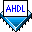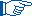## Using Altera-Provided Unparameterized Functions

 See AlsoThe Quartus® II software includes libraries of primitives that are not inherently parameterized. These Quartus II primitives can be used in AHDL to create hierarchical logic designs.

There are two ways to use (that is, insert an instance of) an unparameterized function in AHDL:

• Declare a variable for the function, that is, an instance name, in an Instance Declaration in the Variable Section, and use ports of the instance of the function in the Logic Section.

• Use an in-line logic function reference in the Logic Section of the Text Design File (.tdf).Instance Declarations provide named nodes that are useful for entering resource assignments and simulating a project. In contrast, when an in-line logic function reference does not define an instance name, the instance's node names—which are based on the instance name—may change as the project logic changes. Node names do not change for an in-line logic function reference that defines an instance name.

Function Prototypes for Quartus II primitives are built into the Quartus II software. Therefore, when implementing Quartus II primitives in AHDL, you do not need to declare Function Prototypes using Include Statements.

In the example below, an Instance Declaration creates an instance of a DFF and a TRI primitive.

```SUBDESIGN example1
(
data, clock      : INPUT;
clearn, presetn  : INPUT;
a, b             : INPUT;
q_out, t_out     : OUTPUT;
)
VARIABLE
dff1 : dff;
tri1 : tri;
BEGIN
dff1.d = data;
dff1.clk = clock;
dff1.clrn = clearn;
dff1.prn = presetn;
q_out = dff1.q;
tri1.in = b;
tri1.oe = a;
t_out = tri.out;
END;```

Instance Declarations in the Variable section declare the variables `dff1` and `tri1` as instances of the DFF and TRI primitives, respectively. The ports of the primitives, which are in the format <instance name>`.`<port name>, are assigned values in the Boolean equations in the Logic Section.The help topic describing an Altera-provided function shows the Function Prototype for that function.

The example2.tdf file shown below has the same functionality as example1.tdf, but creates instances of the DFF and TRI primitives with in-line logic function references:

```SUBDESIGN example1
(
data, clock      : INPUT;
clearn, presetn  : INPUT;
a, b             : INPUT;
q_out, t_out     : OUTPUT;
)
BEGIN
q_out = dff1: dff (data, clock, clearn, presetn);
t_out = tri1: tri (.oe = a, .in = b);
END;```

The in-line logic function reference for the DFF and TRI primitives appear in the Boolean equations in the Logic Section. The in-line logic function reference for `dff1` uses positional port association, whereas the in-line logic function reference for `tri1` uses named port association. When positional port association is used, the order of ports is important because there is a one-to-one correspondence between the order of the ports in the Function Prototype and the ports defined in the Logic Section.Because primitives do not necessarily have default values for unconnected inputs, you must ensure that all required ports are connected.